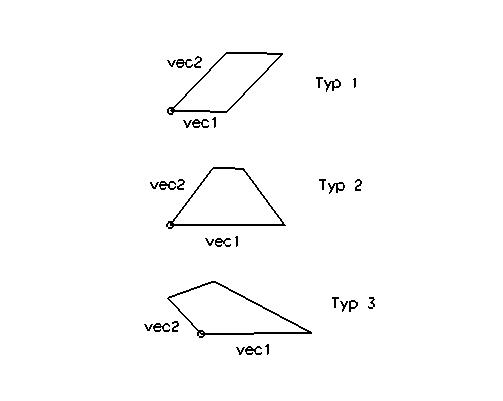```

Shortcut key is S (Spline)
Polygon
Ellipse
Clothoid (spiral-form curve)
Contour (compound curve)

____________________________________________________________________________

S Ellipse Center,AxisEndPoint,Point
Ellipse from center, end point main axis, point on ellipse.

Center               centerpoint
EndPoint MajorAxis   endpoint of the main axis
Point on Ell.        a point on the ellipse

Example:
S1=ELL P(100 0) P(150 0) P(100 20)

S Ellipse Center Axes [EndPoints]   ELL
Limited ellipse from center, vector main axis, vector to point on ellipse.

Center               centerpoint
MajorAxis (VEC)      Vector to the endpoint of the main axis
Axis (VEC)           Vector to a point on the ellipse
[StartPoint]         Optional: startpoint of ellipse.
[EndPoint]           Optional: endpoint

Example:
# Vectors + length and endpoints
S1=ELL P(0 0) D(DX 100) D(DY 80) P(100 0) P(-100 0)

S Polygon < Points...                      POL
Polygon from points

2D-Polygon from points:
S# = POL2, 2D-point1, 2D-point2, <2D-point3, ...2D-pointN>

Example:
P20 = 10.10
S20 = POL2, P20, P(10.0) P(20.10) P(30.30)

3D-Polygon from points:
S#= POL, point1, point2, <point3, ...pointN>

Example:
P20 = P(10.10)
P21 = P(20,20,15)
P22 = P(25.20)
S24 = POL P20 P21 P22 P(30,12,0) P(30,10,10) P(40,30,10)

Quadrilateral from point and 2 vectors or points:
CornerPoint          lower left point
Vec/Pt first side    horizontal vector or point;
Vec/Pt last side     vertical vector or point;
[Type]               Typ 1 (default) for parallelogram, rectangle, square
Typ 2 for trapezium
Typ 3 for rhombus, kiteExample:
# parallelogram, horizontal, length 100; vertical length 12
S20 = REC P(100 -100 0) D(50 0 0) D(DY 32)
# trapezium
S21=REC P(100 0 0) D(100 0 0) D(20 60 0) MOD(2)
# kite
S22=REC P(100 100 0) D(100 0 0) D(-20 60 0) MOD(3)

S BSpline < Points...                      BSP
B-Spline from points
S-bsp = BSP {points} [,degree] [,CTRL]
CTRL - whether the defined points are transit points or check points.

Example:
P20 = P(78.9)
P21 = P(66.28)
P22 = P(44,9,25)
P23 = P(9.12)
P24 = P(6.34)
# B-Spline from Points
S20 = BSP, P20, P21, P22, P23, P24

Tangential to a line:  (CTRL must be active !)
The spline is tangential to the lines defined by the first two and
last two points.

Example:
P21=P(-737.37375 -42.08754 0)
L20=P21 P(-13.468014 335.016819 0)
P22=P(L20 0.5)
L21=P(-457.912466 -159.932652 0) P(390.572397 -149.831643 0)
S20=BSP  P22 P(L20 0.6) P(-70.7 119 0) P(-67 -58 0) P(L21 0.6) P(L21 0.7) CTRL
P23=P(L21 MOD(2))

S BSpline < convert & join objects                    BSP1
Convert and connect one or more objects into a B-Spline curve,
with or without rounding.

Input elements:  Points, lines, arcs,
polygons, ellipses, clothoids or B-Spline-curves.

smoothFactor:     negativ value: degree 1 (polygon)
positive value: degree 2 or 3;
higher values - more controlpoints

Example:
# convert polygon into B-Spline-curve
S21=BSP1 S20
# Connect Linie20 and Linie21 to a curve with rounding.
S20 = BSP1 L20 L21 0.1

S Clothoid (spiral-form curve)             CLOT
Clothoid spiral-form curve: generate (a planar spiral through Fresnel-Integral).
StartPoint                  starting point
StartVector                 launch direction (vector or angle)
Angle                       difference angle from direction towards the end point, positive is clockwise (CW), negative is counter-clockwise (CCW).
[Z-Axis]                    normal vector, optional.

Auxiliary functions to create a clothoidal spiral-form curve
Creation of the start point with "PT cartes"
Select the end of the previous element.
Generating the start vector "VEC tangent"
Select the end of the previous element and the previous element. With "OK", the discharge (outlet) vector will be generated.

Example:
S20 = CLOT P(0 0 0) ANG(0) ANG(30) 0 10

Export of a clothoidal spiral-form curve:
DXF: Issue as POLYLINE.
IGES: Issue as Entity 106 (Copious Data, Form 12 = 3D polygon).

S Contour(CCV) <- PT/LN/CIR/CRV            CCV
Contour ("Composite (compound, concatenated) curve" - CCV)
A contour consists of the elements of points, lines, circles, curves.
The outline should start at a point and end at a point.
The rotational direction (CW or CCW) is defined following circles and curves.

Lines and arcs are automatically connected with normal elements;
Points are directly connected;
Intersections between elements are automatically formed.

Format:
S# = CCV {contour elements}
Example:
C20 = P(39.26) VAL (22)
S20 = CCV P(7.25) C20 CW P(35.58)

A 2D composite curve from 2D polygon
A 2D composite curve consists of circles/lines

S# = CCV2, 2D polygon, tolerance

Example:
P20 = P(78.9)
P21 = P(66.28)
P22 = P(44,9,25)
P23 = P(9.12)
P24 = P(6.34)
# Poly from Points
DRAW OFF
S20 = POL, P20, P21, P22, P23, P24
# BSpline from Poly
DRAW ON
S21 = BSP, S20, 2
# Poly from BSpline
DRAW OFF
S22 = POL, S21, 0.05
# 2DPoly from Poly
DRAW OFF
S23 = POL2 S22 R22 0.05
# 2DCircle/Line from 2DPoly
DRAW ON
S24 = CCV2 S23 0.05

```